## If you weigh 690 N on the earth, what would be your weight on the surface of a neutron star that has the same mass as our sun and a diameter

Question

If you weigh 690 N on the earth, what would be your weight on the surface of a neutron star that has the same mass as our sun and a diameter of 15.0 km ? Take the mass of the sun to be ms = 1.99×1030 kg , the gravitational constant to be G = 6.67×10−11 N⋅m2/kg2 , and the free-fall acceleration at the earth’s surface to be g = 9.8 m/s2 . Express your weight wstar in newtons.

in progress 0
2 months 2021-08-17T02:06:09+00:00 1 Answers 8 views 0

W’ = 1.66 x 10¹⁴ N

Explanation:

First, we will calculate the mass: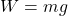where,

W = weight on earth = 690 N

m = mass = ?

g = acceleration due to gravity on earth = 9.8 m/s²

Therefore,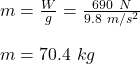Now, we will calculate the value of g on the neutron star: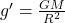where,

g’ = acceleration due to gravity on the surface of the neutron star = ?

G = Gravitational Constant = 6.67 x 10⁻¹¹ N.m²/kg²

M = Mass of the Neutron Star = 1.99 x 10³⁰ kg

R = Radius of the Neutron Star = 15 km/2 = 7.5 km = 7500 m

Therefore,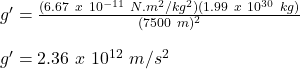Therefore, the weight on the surface of the neutron star will be: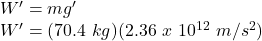W’ = 1.66 x 10¹⁴ N